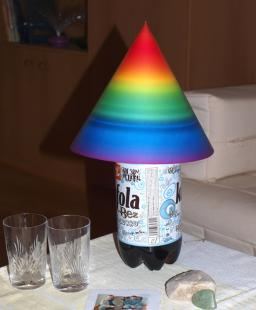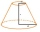# Axial cut

The cone surface is 388.84 cm2, the axial cut is an equilateral triangle. Find the cone volume.

V =  480.6611 cm3

### Step-by-step explanation:Did you find an error or inaccuracy? Feel free to write us. Thank you!Tips to related online calculators
Do you want to convert length units?
Tip: Our volume units converter will help you with the conversion of volume units.
Pythagorean theorem is the base for the right triangle calculator.

#### You need to know the following knowledge to solve this word math problem:

We encourage you to watch this tutorial video on this math problem:

## Related math problems and questions:

• Axial sectionThe axial section of the cone is an equilateral triangle with area 168 cm2. Calculate the volume of the cone.
• Axial sectionCalculate the volume and surface of a cone whose axial section is an equilateral triangle with side length a = 18cm.
• Axial section of the coneThe axial section of the cone is an isosceles triangle in which the ratio of cone diameter to cone side is 2: 3. Calculate its volume if you know its area is 314 cm square.
• Axial cut of a rectangleCalculate the volume and surface of the cylinder whose axial cut is a rectangle 15 cm wide with a diagonal of 25 cm long.
• Surface areaThe volume of a cone is 1000 cm3 and the content area of the axis cut is 100 cm2. Calculate the surface area of the cone.
• Cone - from volume surface areaThe volume of the rotating cone is 1,018.87 dm3, and its height is 120 cm. What is the surface area of the cone?
• Cone sideCalculate the volume and area of the cone whose height is 10 cm and the axial section of the cone has an angle of 30 degrees between height and the cone side.
• Rotary bodiesThe rotating cone and the rotary cylinder have the same volume of 180 cm3 and the same height v = 15 cm. Which of these two bodies has a larger surface area?
• Surface of the coneCalculate the surface of the cone if its height is 8 cm and the volume is 301.44 cm3.
• Truncated coneFind the volume and surface area of the truncated cone if r1 = 12 cm, r2 = 5 cm and side s = 10 cm.
• Lateral surface areaThe ratio of the area of the base of the rotary cone to its lateral surface area is 3: 5. Calculate the surface and volume of the cone, if its height v = 4 cm.
• Frustrum - volume, areaCalculate the surface and volume of the truncated cone, the radius of the smaller figure is 4 cm, the height of the cone is 4 cm and the side of the truncated cone is 5 cm.
• Cone containerRotary cone-shaped container has a volume 1000 cubic cm and a height 12 cm. Calculate how much metal we need for making this package.
• Triangular prism,The regular triangular prism, whose edges are identical, has a surface of 2514 cm ^ 2 (square). Find the volume of this body in cm3 (l).
• Cube diagonalsDetermine the volume and surface area of the cube if you know the length of the body diagonal u = 216 cm.
• Rotating coneFind the rotating cone's surface and volume if its side is 150 mm long and the circumference of the base is 43.96 cm.
• Triangular prism - regularThe regular triangular prism is 7 cm high. Its base is an equilateral triangle whose height is 3 cm. Calculate the surface and volume of this prism.